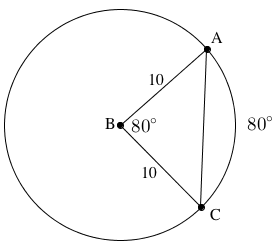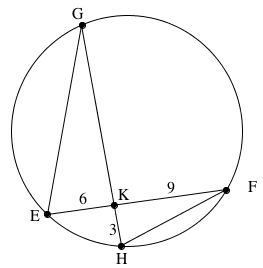### Home > GC > Chapter 10 > Lesson 10.2.3 > Problem10-85

10-85.

Review circle relationships as you answer the questions below.

1. On your paper, draw a diagram of $⊙B$ with $\overarc{ A C }$. If $m\overarc{AC}=80°$ and the length of the radius of $⊙B$ is $10$, find the length of chord $\overline{ A C }$.

According to the Law of Cosines,
$b^2=a^2+c^2−2ab\cos\left(\text{B}\right)$
Therefore, $b^2=10^2+10-2\left(10\right)\left(10\right)\cos\left(80°\right)$1. Now draw a diagram of a circle with two chords, $\overline{EF}$ and $\overline{GH}$, that intersect at point $K$. If $EF=15$, $EK=6$, and $HK=3$, what is $GK$?

Remember that intersecting chords create similar triangles, and that the corresponding sides of similar triangles have equal ratios.

$\frac{9}{3}=\frac{x}{6}$